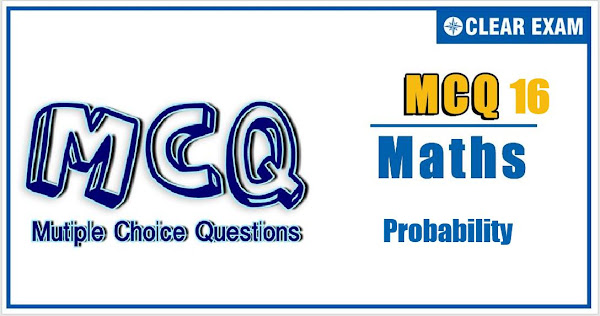## Probability Quiz-16

Probability is an important topic in JEE advanced examination. In this exam, probability carries weightage of 7% of questions. With focused practice good marks can be fetched from this topic .

Q1. Statement 1: If a fair coin is tossed 15 times, then the probability of getting head as many times in the first ten throws as in the last five is 3003/32768
Statement 2: Sum of the series mcrnc0 +mcr-1nc1+...+mc0ncr=m+ncr
•  Statement 1 is True, Statement 2 is True; Statement 2 is correct explanation for Statement 1
•  Statement 1 is True, Statement 2 is True; Statement 2 is not correct explanation for Statement 1
•  Statement 1 is True, Statement 2 is False
•  Statement 1 is False, Statement 2 is True
Q2.Let H1,H2,…,Hn be mutually exclusive events with P(Hi )>0,i=1,2,…,n. Let E be any other event with 0<P(E)<1. Statement 1: P( Hi/E)> P(E/Hi)∙P(Hi) for i=1,2,…,n
Statement 2:•  Statement 1 is True, Statement 2 is True; Statement 2 is correct explanation for Statement 1
•  Statement 1 is True, Statement 2 is True; Statement 2 is not correct explanation for Statement 1
•  Statement 1 is True, Statement 2 is False
•  Statement 1 is False, Statement 2 is True
Q3.  A fair die is rolled once
Statement 1: The probability of getting a composite number is 1/3
Statement 2: There are three possibilities for the obtained number: (i) the number is a prime number, (ii) the number is a composite number and (iii) the number is 1. Hence probability of getting a prime number is 1/3
•  Statement 1 is True, Statement 2 is True; Statement 2 is correct explanation for Statement 1
•  Statement 1 is True, Statement 2 is True; Statement 2 is not correct explanation for Statement 1
•  Statement 1 is True, Statement 2 is False
•  Statement 1 is False, Statement 2 is True

Q4.  If a particular experiment be given n(a finite) independent trials. If the probability of success in one trial (say) p ∴ We get probability of failure, q=(1-p) The probability of r success in n trials =ncr pr qn-r On the basis of above information, answer the following question:
The probability of man hitting a target in one fire is 1/4. At least n times he must fire at the target that the chances of hitting the target at least once will exceed 2/3, then n is:
•  2
•  4
•  6
•  8
Q5.There are n urns each containing (n+1) balls such that the ith urn contains `i' white balls and (n+1-i) red balls. Let u_i be the event of selecting ith urn, i=1,2,3,…,n and W deontes the event of getting white balls. On the basis of above information,answer the following question
P(ui )∝i,where i=1,2,3….,n,then lim(n→∞)⁡〖P(W)〗 is equal to
•  1
•  2/3
•  1/4
•  3/4
Q6. A is set containing 10 elements. A subset P of A is chosen at random and the set A is reconstructed by replacing the elements of P. Another subset Q of A is now chosen at random. Then, the probability if. On the basis of the above information, answer the following questions.
P∪Q=A, is:
•  (1/2)10
•  (2/3)10
• (3/4)10
•  (2/5)10
Q7. In a class of 10 students, probability of exactly i students passing an examination is directly proportional to i^2. Then answer the following question.
The probability that exactly 5 students passing an examination is:
•  1/11
•  5/77
•  25/77
•  10/77
Q8.A shopping mall is running a scheme: ‘Each packet of detergent “SURE” contains a coupon which bears letter of the word, “SURF”, if a person buys at least four packets of detergent “SURF”, and produce all the letters of the word “SURF”, then he gets one free packet of detergent
If a person buys 8 such packets at a time, then number of different combinations of coupon he has:
•  48
•  84
•  11c3
•  12c4
Q9.In an objective paper, there are two sections of 10 questions each. For ‘section 1’, each question has 5 options and only one option is correct and ‘section 2’ has 4 options with multiple answers and marks for a question in this section is awarded only if he ticks all correct answers. Marks for each questions in ‘section 1’ is and in ‘section 2’ is 3. (There is no negative marking)
If a candidate attempts only tw3o questions by guessing, one from ‘section 1’ and one from ‘section 2’, the probability that he scores in both questions is:
•  74/75
•  1/25
•  1/15
•  1/75
Q10. There are two die A and B both having six faces. Die A has three faces marked with 1, two faces marked with 2 and one face marked with 3. Die B has one face marked with 1, two faces marked with 2 and three faces marked with 3. Both dices are thrown randomly once. If E be the event of getting sum of the numbers appearing on top faces equal to x and let P(E) be the probability of even E, then
P(E) is maximum when x equal to:

•  5
•  3
•  4
• 6#### Written by: AUTHORNAME

AUTHORDESCRIPTION## Want to know more

Please fill in the details below:

## Latest NEET Articles\$type=three\$c=3\$author=hide\$comment=hide\$rm=hide\$date=hide\$snippet=hide

Name

ltr
item
BEST NEET COACHING CENTER | BEST IIT JEE COACHING INSTITUTE | BEST NEET & IIT JEE COACHING: Probability-Quiz-16
Probability-Quiz-16
https://1.bp.blogspot.com/-S2TRNnVgKN0/YN3pDq1RrsI/AAAAAAAAAWw/_Rz-IpGXDZMKJkKgW8tB5FlfdqTFsGdZACPcBGAYYCw/s600/p16.JPG
https://1.bp.blogspot.com/-S2TRNnVgKN0/YN3pDq1RrsI/AAAAAAAAAWw/_Rz-IpGXDZMKJkKgW8tB5FlfdqTFsGdZACPcBGAYYCw/s72-c/p16.JPG
BEST NEET COACHING CENTER | BEST IIT JEE COACHING INSTITUTE | BEST NEET & IIT JEE COACHING
https://www.cleariitmedical.com/2021/07/probability-quiz-16.html
https://www.cleariitmedical.com/
https://www.cleariitmedical.com/
https://www.cleariitmedical.com/2021/07/probability-quiz-16.html
true
7783647550433378923
UTF-8

STAY CONNECTED